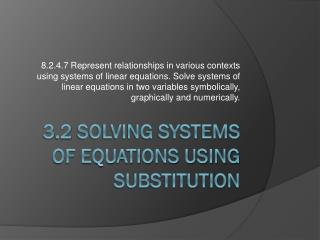DownloadDownload Presentation3 .2 Solving Systems of Equations using Substitution

# 3 .2 Solving Systems of Equations using Substitution

Télécharger la présentation## 3 .2 Solving Systems of Equations using Substitution

- - - - - - - - - - - - - - - - - - - - - - - - - - - E N D - - - - - - - - - - - - - - - - - - - - - - - - - - -
##### Presentation Transcript

1. 8.2.4.7 Represent relationships in various contexts using systems of linear equations. Solve systems of linear equations in two variables symbolically, graphically and numerically. 3.2 Solving Systems of Equations using Substitution

2. Guiding Question: How can I solve a system of equations non-graphically? • Lesson Objective: I will be able to solve a system of equations by non-graphical methods. • How do I solve by substitution? • Solve 1 of the equations for one of the variables • Substitute into the other equation. • Solve for the variable • Substitute the solution into one of the equations. • Solve for the other variable.

3. Guiding Question: How can I solve a system of equations non-graphically? • How do I solve by substitution? • Solve 1 of the equations for one of the variables • Substitute into the other equation. • Solve for the variable • Substitute the solution into one of the equations. • Solve for the other variable. Solve this system Solve this system

4. Guiding Question: How can I solve a system of equations non-graphically? • Solve this system • 2 other possibilities • If an answer is always true (ex. 7 = 7), that means there are infinite solutions. • If an answer is always false (ex. 2 = 8), that means there is no solution.

5. Guiding Question: How can I solve a system of equations non-graphically? • Assignment: “Herd of Buffalo”